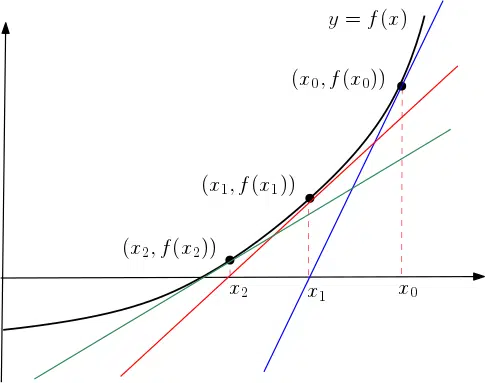# Numerical Methods in A-Level Mathematics

## Numerical Methods

When we cannot solve an equation, for example, using the normal analytical techniques we often use Numerical Methods. This usually requires the use of computers but at A-Level Maths we learn the fundamentals of these processes by doing the calculations by hand (or with a calculator). Recall that we first saw numerical methods in GCSE Maths when using iteration.We do not study Numerical Methods in AS-Maths. However, in Year 2, we take a look at various numerical methods for finding roots. We also explore ways of estimating  the area beneath graphs which up until now we have found using definite integrals.

• (not covered in AS Maths)